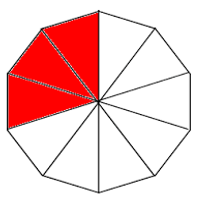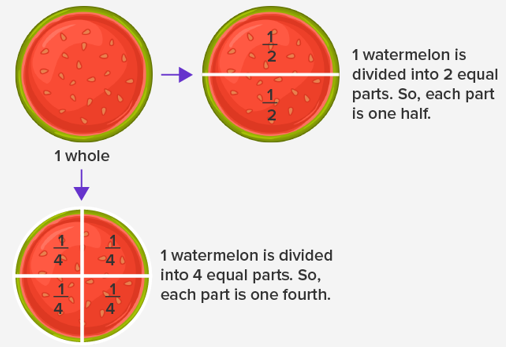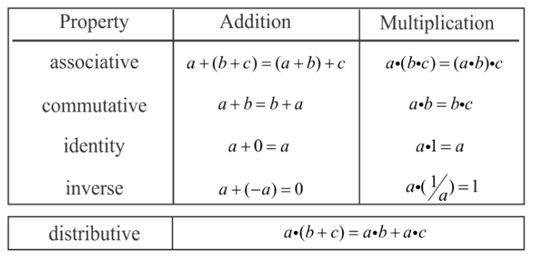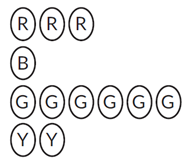Math Concepts
Fractions: Operations and Properties
13.9k views

 1 What are Fractions? 2 Types of Fractions 3 Equivalent Fraction 4 Operations on Fractions 5 Comparison of Fractions 6 Properties of Fractions 7 Examples of properties of fractions 8 Conversion of Fraction to Decimal numbers 9 Practice Questions 10 Answers with explanation 11 Learning outcomes 12 About Cuemath 13 Frequently Asked Questions (FAQs) on Fractions 14 External References

29 January 2021

## What are Fractions?

The term Fraction is derived from the Latin language word “Fractus, “ which means broken. A fraction is defined as part of a group or of a region.

Examples of fraction:In the above figure, a decagon is divided into ten equal parts. Out of ten parts, only three parts are shaded in red. The representation of this shaded portion is known as "Fraction." A Fraction comprises two parts, namely the Numerator and the Denominator. Here the fraction is denoted as $$\frac{3}{10},$$ where 3 is the numerator (the upper part), and 10 is the denominator (the lower part.)

The following figure illustrates the concept of fractions more clearly.### Fractions: Operations and Properties-PDF

Fractions are a part of something. In this article, we will discuss different types of fractions, arithmetic operations on fractions, comparison of fractions, and properties of fractions Here is a downloadable PDF to explore more.

## Types of Fractions

There are three types of fractions.

1. Proper fractions - In a proper fraction numerator is smaller than the denominator.

Example: $$\frac{5}{7}$$

2. Improper Fractions: In an improper fraction, the numerator is greater than the d

Example: $$\frac{13}{10}$$

3. Mixed Fractions: It is a combination of whole and part.

Example: $$1\frac{1}{4}$$

## Equivalent Fraction

In these fractions, although the numerator and denominator are different they have the same value.

For example:

$\frac{3}{5}=\frac{6}{10}=\frac{9}{15}$

In the above example, the fractions $$\frac{6}{10}$$ and $$\frac{9}{15},$$ when reduced to its simpler form it gives $$\frac{3}{5}$$.

So, all the above fractions are said to be equivalent fractions.

## Operations on Fractions

In this topic, we will see some examples of each arithmetic operation: addition, subtraction, multiplication, and division.

Case 1: If the denominators are the same for given fractions, we can directly add the numerators of fractions. And these fractions with the same denominators are called “Like Fractions.”

Example:

$\frac{2}{7}+\frac{3}{7}=\frac{5}{7}$

Case 2: If the denominators are different, the fractions are called, Unlike fractions. In this case, we can not add the numerators directly. First, we need to make the fractions like fractions (Same denominators). Conversion of Unlike fractions to Like Fractions can be done using one of the two methods shown below:

If the denominators are not the same for given fractions

Step 1: Find out the LCM of both denominators.

Step 2: Using the LCM, we could make the Unlike fractions to Like fractions, and then the numerators are simplified.

Example:

$\frac{2}{3}+\frac{5}{7}$

In this example LCM $$= 21$$

$\frac{2 \times 7}{3 \times 7}+\frac{5 \times 3}{7 \times 3}=\frac{14}{21}+\frac{15}{21}=\frac{14+15}{21}=\frac{29}{21}$

Method 2

Step 1:  In this method, we cross multiply the numerator of the first fraction to the denominator of the second fraction and numerator of the second fraction to the denominator of the first fraction.

Step 2: Then multiply both denominators.

In either way, the answer will be the same.

Example:

\begin{align}&\frac{2}{3}+\frac{5}{7} \\[6pt]&\frac{(2 \times 7)+(5 \times 3)}{(3 \times 7)}=\frac{29}{21}\end{align}

Case 3: The addition of mixed fractions can be done in two ways.

Method 1:

Step 1: Add the Wholes and fractions separately as shown.

Step 2: If the fractions to be added are Unlike fractions, add them by finding the LCM of the denominators or by using the cross multiplication method, as shown in Case 2.

Example:

\begin{align}1 \frac{2}{3}+3 \frac{1}{4}& \\[6pt]&=(1+3)\left(\frac{2}{3}+\frac{1}{4}\right) \\[6pt]&=4 \frac{11}{12}\end{align}

Method 2:

In this method,

Step 1: Convert mixed fraction into an improper fraction as shown in the example below.

Step 2: Add them by following the above-discussed methods.

Example:

\begin{align}1 \frac{2}{3}+3 \frac{1}{4} \\[6pt]&1 \frac{2}{3}=\frac{(3 \times 1)+2}{3}=\frac{5}{3} \\[6pt]& 3 \frac{1}{4}=\frac{(3 \times 4)+1}{4}=\frac{13}{4}\end{align}

Add $$\frac{5}{3}, \;\frac{13}{4}$$

$\frac{5}{3}+\frac{13}{4}=\frac{20+39}{12}=\frac{59}{12}=4 \frac{11}{12}$

### Subtractions of Fractions

The subtraction of fractions is similar to the addition of fractions as in the above examples. Instead of addition, we need to subtract at the relevant places.

Case 1: In the case of Like fractions (fractions with the same denominators), we can directly subtract the numerators of fractions.

Example:

$\frac{10}{7}-\frac{2}{7}=\frac{5}{7}$

Case 2: Unlike fractions, we use two different methods, either by taking the LCM of the denominators and making it into a Like fraction and then subtracting it or by cross multiplying. Both the methods are shown with examples below:

Method 1:

Step 1: Find out the LCM of both denominators.

Step 2: Using the LCM, we could make the Unlike fractions to Like fractions, and then the numerators are simplified.

Example:

$\frac{10}{3}-\frac{5}{7}$

In this example LCM $$= 21$$

$\frac{10 \times 7}{3 \times 7}-\frac{5 \times 3}{7 \times 3}=\frac{70}{21}-\frac{15}{21}=\frac{55}{21}$

Method 2

Step 1: Cross multiply the numerator of the first fraction to the denominator of the second fraction and numerator of the second fraction to the denominator of the first fraction.

Step 2: Then Multiply both denominators.

Example:

\begin{align}\frac{10}{3}-\frac{5}{7}&\\[6pt]&\frac{(10 \times 7)-(5 \times 3)}{(3 \times 7)}=\frac{55}{21} \end{align}

Case 3: Subtraction of mixed fractions

Method 1:

In the case of the subtraction of mixed fractions, first, we have to separate the whole part and the fractional part. And then, we can subtract them as per the method discussed above if denominators are different.

Example:

\begin{align}3 \frac{2}{3}-1 \frac{1}{4}& \\[6pt]&=(3-1)\left(\frac{2}{3}-\frac{1}{4}\right) \\[6pt]&=2 \frac{5}{12}\end{align}

Method 2:

In this method of subtraction of mixed fractions, we have to convert a mixed fraction into an improper fraction.

Example:

\begin{align}3 \frac{2}{3}-1 \frac{1}{4}& \\[6pt]&=\frac{(3 \times 3)+2}{3}-\frac{(1 \times 4)+1}{4}\\[6pt]&=\frac{11}{3}-\frac{5}{4} \\[6pt]&=\frac{(11 \times 4)-(5 \times 3)}{12}=\frac{39}{12}\\[6pt]&=2 \frac{5}{12}\end{align}

### Multiplication of Fractions

The multiplication of fractions is quite simple. We have to multiply numerators of given fractions and then multiply denominators of the given fractions. If required we could convert the fraction into its simplest form or the reduced form.

Examples:

1. $$\frac{4}{5} \times \frac{7}{9}=\frac{4 \times 7}{5 \times 9}=\frac{28}{45}$$

2. $$\frac{3}{4} \times 8=\frac{3 \times 8}{4}=\frac{24}{4}=6$$

3. $$1 \frac{2}{3} \times 3 \frac{3}{5}=\frac{5}{3} \times \frac{18}{5}=\frac{5 \times 18}{3 \times 5}=\frac{90}{15}=\frac{18}{3}$$ (hence $$\frac{18}{3}$$ is the reduced form of $$\frac{90}{5}$$ )

### Division of Fractions

The division of fractions includes the following steps

Step 1: Here we need to include the concept of reciprocal of fractions which means the inverse of the given fraction.

Example:

1.  Reciprocal of $$\frac{2}{3}$$ is $$\frac{3}{2}$$

2.  A reciprocal of 5 is $$\frac{1}{5}$$

Step 2: Multiply the first fraction with the reciprocal of the first fraction.

Examples of the division of fractions

1. $$\frac{3}{5} \div \frac{5}{7}=\frac{3}{5} \times \frac{7}{5}=\frac{21}{25}$$

2. $$5\div \frac{5}{7}=5 \times \frac{7}{5}=\frac{35}{5}=7$$ (Simplifed form)

3. $$\frac{4}{5} \div 6=\frac{4}{5} \times \frac{1}{6}=\frac{2}{15}$$ (Reduced form)

## Comparison of Fractions

The larger the numerator the larger the fraction, and the larger the denominator the smaller the fraction.

If the denominators are the same, the fraction with a larger numerator is larger and if the numerators are the same, the fraction with the larger denominator is smaller.

Case 1: When denominators are the same but numerators are different.

In this example, the fraction having a greater numerator is the greater fraction.

Example:

Compare $$\frac{6}{8}$$ and $$\frac{7}{8}$$

$$\frac{6}{8}<\frac{7}{8}$$

Case 2: When numerators are the same but denominators are different.

In this example, the fraction having a lesser denominator is the greater fraction.

Example:

Compare $$\frac{13}{9}$$ and $$\frac{13}{10}$$

$$\frac{13}{9}>\frac{13}{10}$$

Case 3: When numerators and denominators of the given fractions are different, we have to make the fractions into like fractions by finding the LCM of denominators. The like fractions are then compared as shown above.

Example:

Compare $$\frac{2}{5}$$ and $$\frac{4}{7}$$

LCM of 5 and 7 is 35

\begin{align}&\frac{2 \times 7}{5 \times 7}=\frac{14}{35}\quad \text { and }\quad \frac{4 \times 5}{7 \times 5}=\frac{20}{35} \\[6pt]&\frac{14}{35}<\frac{20}{35}\end{align}

## Properties of Fractions

There are different properties of fractions over multiplication and addition and they are listed in the below table.Each property is explained with examples below.

## Examples of properties of fractions

1. Associative Property

Similar to Numbers and Integers, associative property gets applied in fractions too, over Addition and Multiplication.

Example:

$\frac{1}{2}+\left(\frac{1}{3}+\frac{1}{4}\right)=\left(\frac{1}{2}+\frac{1}{3}\right)+\frac{1}{4}$

In this example, the answer will be on both the LHS and RHS side. Hence we can say that fractions follow the associative property of addition.

Associative Property of Multiplication

Example:

$\frac{2}{5} \times\left(\frac{1}{4} \times \frac{3}{2}\right)=\left(\frac{2}{5} \times \frac{1}{4}\right) \times \frac{3}{2}$

In this example, the answer will be $$\frac{3}{20}$$ on both the LHS and RHS side.

Hence we can say that fractions follow the associative property of addition.

2. Commutative Property

Example:

$\frac{1}{3}+\frac{1}{4}=\frac{1}{4}+\frac{1}{3}$

In this example, the answer is $$\frac{7}{12}$$ on both the LHS and RHS side. Hence we can say that fractions follow the associative property of addition. This property is followed by all types of fractions.

Commutative Property of Multiplication

Example:

$\frac{1}{4} \times \frac{3}{2}=\frac{1}{4} \times \frac{3}{2}$

In this example, the answer is $$\frac{3}{8}$$ on both the LHS and RHS side. Hence we can say that fractions follow the associative property of addition. This property is followed by all types of fractions.

3. Identity Property

Example:

$\frac{4}{5}+0=\frac{4}{5}$

The addition of zero to any fraction gives the fraction itself.

Identity Property of Multiplication

Example:

$\frac{4}{5} \times 0=0$

Any fraction multiplied by zero gives zero.

4. Inverse Property

Example:

$\frac{3}{5}+\left(-\frac{3}{5}\right)=0$

The identity property of addition gives 0.

Identity Property of Multiplication

Example:

$\frac{3}{5} \times \frac{1}{\frac{3}{5}}=1$

Identity property of addition gives 1.

5. Distributive Property

Example:

$\frac{3}{5}\left(\frac{2}{3}+\frac{1}{5}\right)=\left(\frac{3}{5} \times \frac{2}{3}\right)+\left(\frac{3}{5} \times \frac{1}{5}\right)$

In this example, the answer is $$\frac{13}{25}$$ on both the LHS and RHS side. Hence we can say that fractions follow the distributive property of addition.

## Conversion of Fraction to Decimal numbers

Usually, this conversion is done using any of the two methods:

1. Long division method - Dividing the numerator with the denominator.

2. By converting the denominator into a number which is powers of 10. Like 10, 100, 1000, etc. If it is converted it is easy for us to find the decimal number when the fraction has powers of 10 in the denominator.

Examples

1.  $$\frac{5}{12}=0.4166$$

2.   $$\frac{125}{215}=0.581$$

3.  $$\frac{5}{8}=\frac{5 \times 125}{8 \times 125}=\frac{625}{1000}=0.625$$

4.  $$\frac{3}{4}=\frac{3 \times 25}{4 \times 25}=\frac{75}{100}=0.75$$

## Practice Questions

Question 1: The decimal representation of $$2\frac{3}{5}$$ is ______

 (A) 2.5 (B) 2.6 (C) 2.55 (D) 2.50

Question 2: 130.02 + 113.26 – 27.1 – 17.4 = ?

 (A) 180.78 (B) 178.78 (C) 286.78 (D) 198.78

Question 3: The product of two decimals is 33.655. If one of them is 1.27, the other number is ____________.

 (A) 27.5 (B) 26.5 (C) 27.25 (D) 25.75

Question 4: The value of “$$\left[\left(-2 \frac{3}{4}\right)-\left(-1 \frac{3}{4}\right)\right]+\left[\left(-2 \frac{3}{4}\right)-\left(-1 \frac{3}{4}\right)\right]+\ldots$$” up to 30 times is _________.

 (A) –1 (B) 1 (C) 30 (D) –30

Question 5: Simplify: $$222- \left[\frac{1}{3}\{42+(56-\underline{8}+9)\}+108\right]$$

 (A) 87 (B) 195 (C) 89 (D) 159

Question 6: $$123.45 × 67.890 × 1.032$$ is the same as_______.

 (A)  $$1234.5 × 6.789 × 10320$$ (B)  $$1234.5 × 67.89 × 103.2$$ (C)  $$12.345 × 6789.0 × 10.32$$ (D) $$1234.5 × 67.89 × 0.1032$$

Question 7: A farmer has 384 animals, out of which one-third is cows and one-fourth of the cows are dairy cows. How many dairy cows does he have?

 (A) 160 (B) 96 (C) 32 (D) 128

Question 8: Gopal has a bag that contains 3 red, 1 blue, 6 green, and 2 yellow marbles. What fractional part of the bag of marbles is red?(A) $$\frac{1}{12}$$ (B) $$\frac{2}{12}$$ (C) $$\frac{3}{12}$$ (D) $$\frac{6}{12}$$

1. Solution: $$2 \frac{3}{5}=\frac{(2 \times 5)+3}{5}=\frac{13}{5}=2.6$$

Ans: B

2. Solution: $$130.02 + 113.26 – 27.1 – 17.4=198.78$$

Ans: D

3. Solution: Let the other number be x.

\begin{align}1.27 \times X&=33.655 \\[6pt]X&=\frac{33.655}{1.27}=26.5\end{align}

Therefore, the other number is 26.5.

Ans: B

4. Solution: $$\left[\left(-2 \frac{3}{4}\right)-\left(-1 \frac{3}{4}\right)\right]+\left[\left(-2 \frac{3}{4}\right)-\left(-1 \frac{3}{4}\right)\right]+\ldots \ldots \ldots \ldots+30$$ times

\begin{align}{\left[\left(-2 \frac{3}{4}\right)-\left(-1 \frac{3}{4}\right)\right]} &=-1\\[6pt]-1 \times 30&=-30\end{align}
Ans: D

5. Solution: $$222- \left[\frac{1}{3}\{42+(56-\underline{8}+9)\}+108\right]$$

In this example we have to solve the innermost bracket first

\begin{align}222\left[\frac{1}{3}\{81\}+108\right]& \\[6pt]&=222-135\\[6pt]&=87\end{align}

Ans: A

6. Solution: $$123.45 × 67.890 × 1.032=1234.5 × 67.89 × 0.1032$$

L.H.S.$$=123.45 × 67.890 × 1.032 = 8649.213156$$

R.H.S.$$=1234.5 × 67.89 × 0.1032 = 8649.213156$$

Among all the given options only option D is giving the same that is 8649.213156

Ans: D

7. Solution$$\frac{1}{3} \times 384=128$$ is cows and $$\frac{1}{4} \times 128=32$$ Dairy cows

Ans: C

8. Solution: A bag that contains 3 red, 1 blue, 6 green, and 2 yellow marbles

Total number of balls $$=12$$

The fraction of red marbles \begin{align}=\frac{\text{no of balls in red color}}{\text{total number of balls in bag}}=\frac{3}{4}\end{align}

Ans: C

## Learning outcomes

• Identify the number of shaded parts and the number of equals parts in a shape (circle, rectangle).

• Identify a fraction by comparing the number of shaded parts to the total number of parts.

• Write a fraction using mathematical notation and using words.

• Recognize, the value of a fraction is dependent on the number of shaded parts and not on the position of the shaded parts.

• Understanding how to solve addition, subtraction, multiplication, and division of fractions.

Cuemath, a student-friendly mathematics platform, conducts regular Online Classes for academics and skill-development, and their Mental Math App, on both iOS and Android, is a one-stop solution for kids to develop multiple skills. Understand the Cuemath Fee structure and sign up for a free trial.

## What is a fraction? Give examples?

A fraction tells us how many parts of a whole we have a numerator, and a denominator. For example, 1/2 is a fraction.

## How do you know if it's a proper or improper fraction?

Fractions that are less than one are known as proper fractions, and the numerator (is less than the denominator. A fraction with a numerator that is greater than or equal to the denominator is known as an improper fraction. It represents a number greater than or equal to one.

Related Articles